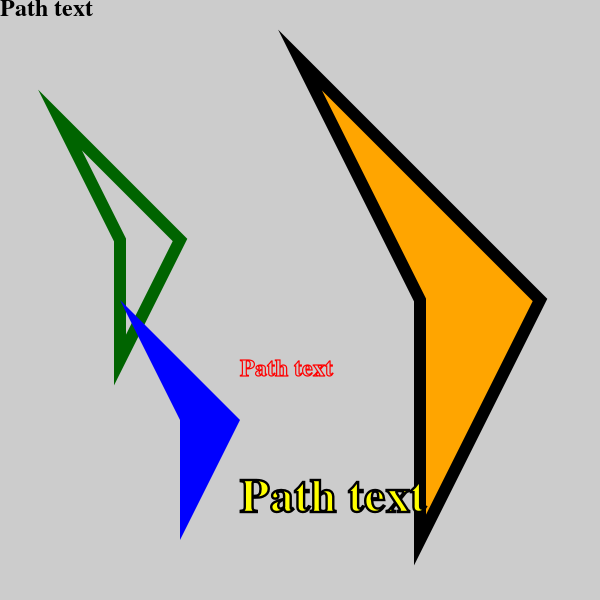# Path objects in generativepy

Martin McBride, 2020-10-04
Tags generativepy tutorial path
Categories generativepy generativepy tutorial

This tutorial covers the basics of using Path objects in generativepy.

## Path example code

Here is a sample Python program for creating various filled and outlined rectangles. The code is explained later in this article:

from generativepy.drawing import make_image, setup
from generativepy.color import Color
from generativepy.geometry import Path, Polygon, Text
import math

def draw(ctx, width, height, frame_no, frame_count):
setup(ctx, width, height, width=5, background=Color(0.8))

# Get a polygon path object
path1 = Polygon(ctx).of_points([(0, 0), (1, 1),
(0.5, 2), (0.5, 1)])\
.path()

# Get a text path object
path2 = Text(ctx).of("Path text", (0, 0))\
.font("Times")\
.size(0.2)\
.align_left()\
.align_top()\
.path()

# Apply the polygon in various places
ctx.save()
ctx.translate(0.5, 1)
Path(ctx).of(path1).stroke(Color('darkgreen'), 0.1)
ctx.restore()

ctx.save()
ctx.translate(1, 2.5)
Path(ctx).of(path1).fill(Color('blue'))
ctx.restore()

ctx.save()
ctx.translate(2.5, 0.5)
ctx.scale(2, 2)
Path(ctx).of(path1).fill(Color('orange')).stroke(Color('black'), 0.05)
ctx.restore()

# Apply the text in various places
ctx.save()
ctx.translate(0, 0)
Path(ctx).of(path2).fill(Color('black'))
ctx.restore()

ctx.save()
ctx.translate(2, 3)
Path(ctx).of(path2).stroke(Color('red'), 0.01)
ctx.restore()

ctx.save()
ctx.translate(2, 4)
ctx.scale(2, 2)
Path(ctx).of(path2).fill(Color('yellow')).stroke(Color('black'), 0.01)
ctx.restore()

make_image("path-method.png", draw, 600, 600)


Here is the resulting image:## Creating the paths

The first part of the code creates a couple of paths. Here is the first:

    path1 = Polygon(ctx).of_points([(0, 0), (1, 1),
(0.5, 2), (0.5, 1)])\
.path()


In this case, we have created a Polygon in the usual way, but instead of filling or stroking the shape, we call path. This returns a Pycario path object. You can think of the path object as being like a set of instructions for drawing the shape.

This code doesn't actually draw anything, it just saves the drawing instructions in path1.

Here is the second path:

    path2 = Text(ctx).of("Path text", (0, 0))\
.font("Times")\
.size(0.2)\
.align_left()\
.align_top()\
.path()


This time we have created a complex path - some text. But the result is the same, we don't draw anything but the instructions for drawing the text are store in path2.

## Drawing path1

Here is the code that actually draws path1

    ctx.save()
ctx.translate(0.5, 1)
Path(ctx).of(path1).stroke(Color('darkgreen'), 0.1)
ctx.restore()

ctx.save()
ctx.translate(1, 2.5)
Path(ctx).of(path1).fill(Color('blue'))
ctx.restore()

ctx.save()
ctx.translate(2.5, 0.5)
ctx.scale(2, 2)
Path(ctx).of(path1).fill(Color('orange')).stroke(Color('black'), 0.05)
ctx.restore()


In the first section, we save the current context and then call translate. This moves user space by (0.5, 1). See useful context methods for more information about this.

We then use a Path object. This takes the drawing instructions in path1, and adds them to the context ready to be drawn. We then stroke the shape in dark green. If you look at the definition of the polygon that is used to define path1, you will see that the top left corner of the polygon is at (0, 0), but because we have tranlsted user space, the polygon actually gets drawn at (0.5, 1).

Finally we call restore. This sets the context back to the state it was in when save was called, so it effectively undoes the translate.

The second block draws the same shape again, this time at (1, 2.5), and it fills it rather than outlining it.

The third block draws the shape yet again, this time at (2.5, 0.5). In this case we also scale user space by (2, 2), which scales user space in the x and y directions. We fill and outline the shape, and because of the scaling the shape appears twice as big.

As we said before, restore takes us back to the previous save, so in this case it undoes the translate and the scale.

## Drawing path2

Here is the code that draws path2

    ctx.save()
ctx.translate(0, 0)
Path(ctx).of(path2).fill(Color('black'))
ctx.restore()

ctx.save()
ctx.translate(2, 3)
Path(ctx).of(path2).stroke(Color('red'), 0.01)
ctx.restore()

ctx.save()
ctx.translate(2, 4)
ctx.scale(2, 2)
Path(ctx).of(path2).fill(Color('yellow')).stroke(Color('black'), 0.01)
ctx.restore()


This illustrates the use of complex paths. We draw the same text path filled, stroked, and also scaled, filled and stroked.